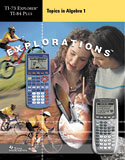## Topics in Algebra 1: Student and Teacher Classroom Materials

### The five chapters in this book describe how to integrate the powerful Calculator Software Application into the Algebra 1 classroom so students can easily review and reinforce selected math concepts.

Tables of Contents

Chapter 1: Number Sense

• Integers
• Rational Numbers
• Real Numbers

• Chapter 2: Linear Equations
• Using Graphs & Tables
• Using Algebra

• Chapter 3: Linear Functions
• Slope with Grid
• Slope Using Coordinates
• Slope as Rate of Change
• Slope-Intercept Form

• Chapter 4: Linear Inequalities: 1-Variable
• Using Graphs &Tables
• Using Algebra

• Chapter 5: Linear Systems
• Using Graphs and Tables
• Using Algebra